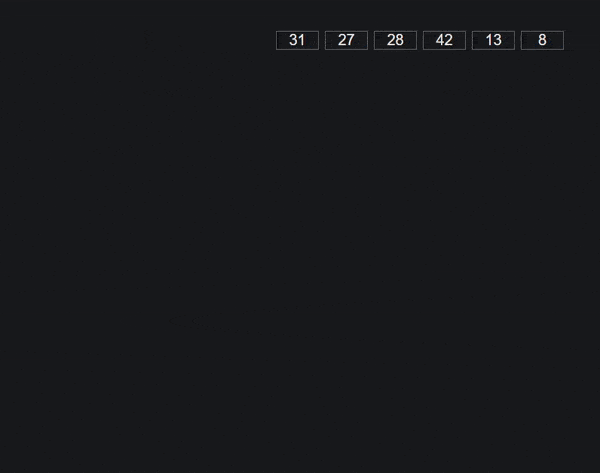# Understanding Radix Sort Through JavaScript

While this tutorial has content that we believe is of great benefit to our community, we have not yet tested or edited it to ensure you have an error-free learning experience. It's on our list, and we're working on it! You can help us out by using the "report an issue" button at the bottom of the tutorial.

Because of the nature of comparison-based sorting, it’s mathematically impossible to improve much beyond `O(nlogn)`, like with merge sort and quick sort. Instead, we can be a bit more clever and avoid comparisons all together to get something closer to `O(n * m)`.

## Prerequisites

A basic understanding of Big O Notation is essential to think about radix sort relative to other algorithms.

## Concept

Radix sort, also known as bucket sort, is one of the oldest sorting algorithms and even pre-exists computers. It was used to sort punched cards back in the 1880s.

It’s based on the idea of having a sub-array, or bucket, for each type of data we need to compare, like A-Z or in our case 0-9. We take the first character/digit in each item, add the whole item to it’s corresponding bucket, then put them back into an array while retaining their new order.

We can then move on to the next character/digit and repeat the process. When an item runs out of characters/digits we’ll add it to the first bucket, since everything else is obviously larger/longer. When we’ve done this as many times as the number of digits/characters of the largest item, our array will have been completely sorted without making any pesky comparisons.Graphic/Animation thanks to VisuAlgo.net

## Practice Data

Since numbers are much simpler, we can practice with an array of them from 1 to 50.

``````const unsortedArr = [31, 27, 28, 42, 13, 8, 11, 30, 17, 41, 15, 43, 1, 36, 9, 16, 20, 35, 48, 37, 7, 26, 34, 21, 22, 6, 29, 32, 49, 10, 12, 19, 24, 38, 5, 14, 44, 40, 3, 50, 46, 25, 18, 33, 47, 4, 45, 39, 23, 2];
``````

## Utilities

Since we’re working with numbers, we want to start with the smallest number place and work up, so we’ll need a way to get each number at an index starting from the right.

The most intuitive way I’ve found is to take the number we want, convert it into a string, and select from it as an array with a negative index. If a number’s not at that index we can just return a zero so it’ll be placed in the front of our sorted array.

``````const getNum = (num, index) => {
const strNum = String(num);
let end = strNum.length - 1;
const foundNum = strNum[end - index];

if (foundNum === undefined) return 0;
else return foundNum;
};

console.log(getNum(4353, 2));
``````

Because we’re working back one digit at a time we need the algorithm to run as many times as the longest number, so if we have an item with 8 digits, it needs to be ran 8 times. Radix sort’s average complexity is `O(n * m)` because it’s the amount of items times the amount of times it needs to be ran.

To get how many times it should run we can search through the array for the largest number, then return its length.

``````const largestNum = arr => {
let largest = "0";

arr.forEach(num => {
const strNum = String(num);

if (strNum.length > largest.length) largest = strNum;
});

return largest.length;
};
``````

Implementation is pretty straight-forward, for every digit place we can use `Array.from` to create 10 empty buckets. For every item they’ll be placed in the corresponding bucket, when that’s done we’ll flatten the array of buckets into a single array to start over with the next character place. When we’ve reached the end of our longest digit our fully sorted array can be returned.

``````const radixSort = arr => {
let maxLength = largestNum(arr);

for (let i = 0; i < maxLength; i++) {
let buckets = Array.from({ length: 10 }, () => []);

for (let j = 0; j < arr.length; j++) {
let num = getNum(arr[j], i);

if (num !== undefined) buckets[num].push(arr[j]);
};
arr = buckets.flat();
};
return arr;
};